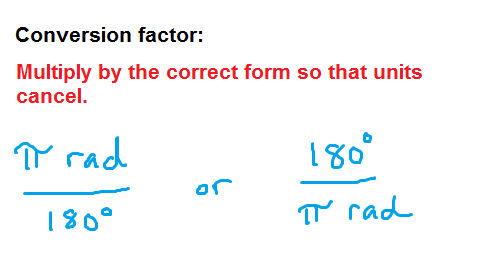﻿ Degrees to radians: converting between these two ways of measuring angles - MathBootCamps

# Degrees to radians: converting between these two ways of measuring angles

To convert from degrees to radians or from radians to degrees, you only have to remember one basic idea: there are 180° in $$\pi$$ radians. This can be used as a conversion factor in any conversion calculation. In the lesson below, we will look at examples of how to apply this idea.

## Examples of converting degrees to radians

The basic idea of any conversion will be to use the following rule.This means that when converting from degrees to radians, you want the degrees to cancel out, leaving radians. Therefore, you will multiply by the following conversion factor:

$$\dfrac{\pi\text{ rad}}{180^{\circ}}$$

### Example

Convert 80° to radians.

### Solution

Multiply 80° by the conversion factor so that degrees cancel.

\begin{align} 80^{\circ} \times \dfrac{\pi\text{ rad}}{180^{\circ}} &= \dfrac{80\pi}{180} \text{ rad} \\ &= \dfrac{4 \times 20\pi}{9 \times 20} \text{ rad} \\ &= \bbox[border: 1px solid black; padding: 2px]{\dfrac{4\pi}{9} \text{ rad}}\end{align}

The only real work is simplifying the resulting fraction, as it is standard to give an exact answer and not a decimal approximation. Let’s look at one more example to be sure it all makes sense.

### Example

Convert 145° to radians.

### Solution

Multiply 145° by the conversion factor so that degrees cancel.

\begin{align}145^{\circ} \times \dfrac{\pi\text{ rad}}{180^{\circ}} &= \dfrac{145\pi}{180} \text{ rad} \\ &= \dfrac{5 \times 29\pi}{5 \times 36} \text{ rad}\\ &= \boxed{\dfrac{29\pi}{36} \text{ rad}}\end{align}

## Examples of converting radians to degrees

The exact same idea holds when going in the reverse direction, where you are taking radians and converting to degrees. Since you want radians to be cancelled out, you will multiply by:

$$\dfrac{180^{\circ}}{\pi \text{ rad}}$$

### Example

Convert $$\dfrac{\pi}{6} \text{ rad}$$ to degrees.

### Solution

Multiply $$\dfrac{\pi}{6} \text{ rad}$$ by the conversion factor so that radians cancel. Notice that $$\pi$$ also cancels.

\begin{align} \dfrac{\pi}{6} \text{ rad} \times \dfrac{180^{\circ}}{\pi \text{ rad}} &= \dfrac{180^{\circ}}{6} \\ &= \bbox[border: 1px solid black; padding: 2px]{30^{\circ}}\end{align}

Note that this is actually one of the common conversions that you should know if you are studying trigonometry.

In the next example, you will see that the answer doesn’t come out quite so nice. To handle this, we will convert to a decimal approximation, which is more common when working with degrees.

### Example

Convert $$\dfrac{3\pi}{7} \text{ rad}$$ to degrees.

### Solution

Multiply $$\dfrac{3\pi}{7} \text{ rad}$$ by the conversion factor so that radians cancel. Again, remember that $$\pi$$ will cancel as well.

\begin{align} \dfrac{3\pi}{7} \text{ rad} \times \dfrac{180^{\circ}}{\pi \text{ rad}} &= \dfrac{180^{\circ} \times 3}{7} \\ &\approx \bbox[border: 1px solid black; padding: 2px]{77.14^{\circ}}\end{align}

Next, we will look at the common radians to degrees conversions you should memorize.

## Common conversions

In trigonometry, there are several common angles that you will want to be comfortable thinking about in terms of degrees or radians. These are shown in the table below.

30° $$\dfrac{\pi}{6} \text{ rad}$$
45° $$\dfrac{\pi}{4} \text{ rad}$$
60° $$\dfrac{\pi}{3} \text{ rad}$$
90° $$\dfrac{\pi}{2} \text{ rad}$$
180° $$\pi \text{ rad}$$
270° $$\dfrac{3\pi}{2} \text{ rad}$$
360° $$2\pi$$

## Continue your study

Now that you have reviewed how to convert between radians and degrees, you can continue your study of trigonometry topics with the following articles.## Subscribe to our Newsletter!

We are always posting new free lessons and adding more study guides, calculator guides, and problem packs.

Sign up to get occasional emails (once every couple or three weeks) letting you know what's new!